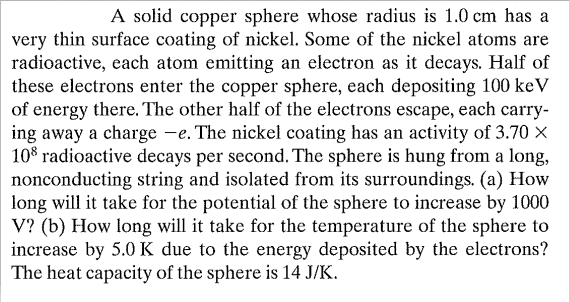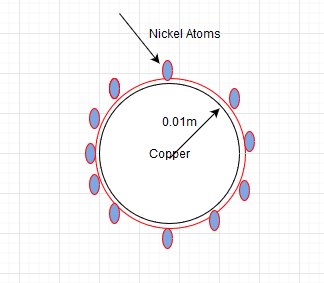# Calcualtion of electric potential and radioactive decay

Physicslearner500039
Homework Statement:
A solid copper sphere whose radius is 1.0 cm has a very thin surface coating of nickel. Some of the nickel atoms are radioactive, each atom emitting an electron as it decays. Half of these electrons enter the copper sphere, each depositing 100 keV of energy there. The other half of the electrons escape, each carrying away a charge -e. The nickel coating has an activity of 3.70 X 10^8 radioactive decays per second. The sphere is hung from a long, nonconducting string and isolated from its surroundings. (a) How long will it take for the potential of the sphere to increase by 1000V? (b) How long will it take for the temperature of the sphere to increase by 5.0 K due to the energy deposited by the electrons? The heat capacity of the sphere is 14 J/K.
Relevant Equations:
NAThis is just a representative diagram to visualizeSurely a very tough one for me to solve. The number of nickel atoms are not mentioned. if the number of decays are ##3.78∗10^8## and with each decay depositing 100keV. The total energy deposited is
##100keV∗3.78∗10^8=6.048∗10^6##
I have to multiple this with the number of atoms to get total energy. Not sure how to proceed further. Please advise.

Last edited:

You don't need to know the number of nickel atoms. It tells you how many decay per second, which is all you need to know. Also, in your calculation of the total energy, is it really a total energy? What are its units? One other thing is that your statement of the heat capacity makes no sense. 1411K makes no sense as a heat capacity. What units should a heat capacity have?

•Physicslearner500039
Physicslearner500039
1411K makes no sense as a heat capacity. What units hould a heat capacity have?
The question was in the attachment
View attachment 1588090631975.png

•